# Class 8.A

Three quarters of class 8.A went skiing. Of those who remained at home one third was ill and the remaining six were on math olympic. How many students have class 8.A?

x =  36

### Step-by-step explanation:

3/4 x + (1-3/4) x/3 + 6 = x

3/4•x + (1-3/4)•x/3 + 6 = x

2x = 72

x = 36

Our simple equation calculator calculates it.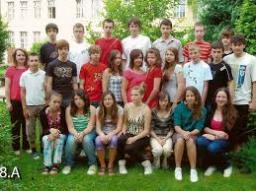Did you find an error or inaccuracy? Feel free to write us. Thank you!Tips to related online calculators
Need help to calculate sum, simplify or multiply fractions? Try our fraction calculator.
Do you have a linear equation or system of equations and looking for its solution? Or do you have a quadratic equation?

## Related math problems and questions:

• English and math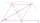1/5 of a class study English, and 3/5 study mathematics. If the class consist of 80 students, how many students study English and mathematics in the class?
• ClassroomOne-eighth of 9th class was interested in studying at a grammar school, at a business academy one sixth, at secondary vocational schools quarter, to SOU one third and the remaining three students were interested in the school of art direction. How many st
• Marks at schoolThere are 30 students in the class. Five students in the class had a mark three triple at the end of the math certificate, and the other students had a mark of one or two. The average mark in all students' mathematics in the class at the end of the year w
• Math classificationIn 3A class are 27 students. One-third got a B in math, and the rest got an A. How many students received a B in math?
• Classs meanClass size is 30 students, math was not worse than sign 2. Determine the number of pupils who were sign 1 if the class had a 1.4 sign average.
• Math test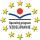In class 6.A are 26 students, and the teacher had managed to give tests to 13 students. 13 testes he gave in 6.5 min. How many pupils still haven't test? How long will it take the teacher to give tests to 43 students?
• Sewing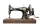The lady cut off one-half of the cloth. She needed three-quarters of this piece to sew a skirt. What part of the original piece of cloth remained?
• Students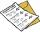The class has 22 students. 2 of which has assesement of 2. Count of assesement of 1 is three times more than count of students with assesement of 3. How many students has assesement of 1 and how many students has assesement of 1.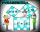Two fifth-graders teams competing in math competitions - in Mathematical Olympiad and Pytagoriade. Of the 33 students competed in at least one of the contest 22 students. Students who competed only in Pytagoriade were twice more than those who just compet
• There 12There are 42 students in the class and 2/3 of them are girls. How may girls are there in the class?
• Dentist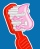Class 5. A participated in a preventive dental examination. A quarter of the pupils found two dental caries, an eighth of the pupils found one caries. Half the class had all their teeth healthy. How many students attend class 5. A if 4 students were missi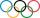Pupils doing research which a winter sport do their classmates most popular. They found that 2/5 of classmates would most like to play hockey, skate prefer 2/9 pupils, 3/10 students prefer skiing and 1/15 classmates don't like any winter sport. What propo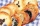A teacher baked 5 dozen cookies for the 16 students in her class. If the teacher divided the cookies equally among her students, how many cookies did she give each student?In a classroom, 1/6 of the students are wearing blue shirts and 2/3 are wearing white shirts. There are 36 students in the class. How many students are wearing a shirt other than blue or white?On the final certificate have one quarter of the class 9A mark "C" of mathematics, seventh mark "C" from the Czech language and two students failed in chemistry. How many students attend class 9A?When asked how many students are in class, Jane said, if we increase the number of students in our class by hundred % and then add half the number of students, we get 100. How many students are in Jane's class?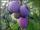In the bag was to total 136 plums. Igor took 3 plums and Mary took 4/7 from the rest. How many plums remained in the bag?